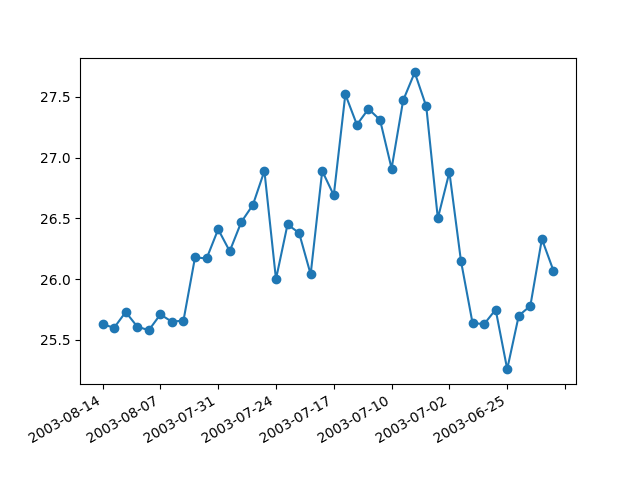Travis-CI:# Date Index Formatter¶

When plotting daily data, a frequent request is to plot the data ignoring skips, e.g., no extra spaces for weekends. This is particularly common in financial time series, when you may have data for M-F and not Sat, Sun and you don’t want gaps in the x axis. The approach is to simply use the integer index for the xdata and a custom tick Formatter to get the appropriate date string for a given index.Out:

```loading /tmp/build_docs/lib/python3.6/site-packages/matplotlib/mpl-data/sample_data/msft.csv
```

```from __future__ import print_function
import numpy as np
from matplotlib.mlab import csv2rec
import matplotlib.pyplot as plt
import matplotlib.cbook as cbook
from matplotlib.ticker import Formatter

datafile = cbook.get_sample_data('msft.csv', asfileobj=False)
r = csv2rec(datafile)[-40:]

class MyFormatter(Formatter):
def __init__(self, dates, fmt='%Y-%m-%d'):
self.dates = dates
self.fmt = fmt

def __call__(self, x, pos=0):
'Return the label for time x at position pos'
ind = int(np.round(x))
if ind >= len(self.dates) or ind < 0:
return ''

return self.dates[ind].strftime(self.fmt)

formatter = MyFormatter(r.date)

fig, ax = plt.subplots()
ax.xaxis.set_major_formatter(formatter)
ax.plot(np.arange(len(r)), r.close, 'o-')
fig.autofmt_xdate()
plt.show()
```

Total running time of the script: ( 0 minutes 0.032 seconds)

Gallery generated by Sphinx-Gallery